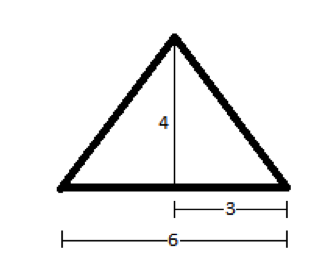# PSAT Math : How to find the length of the side of an acute / obtuse isosceles triangle

## Example Questions

### Example Question #11 : Isosceles Triangles

An isosceles triangle has an area of 12. If the ratio of the base to the height is 3:2, what is the length of the two equal sides?

4

3√3

4√3

5

6

5

Explanation:

Area of a triangle is ½ x base x height. Since base:height = 3:2, base = 1.5 height.  Area = 12 = ½ x 1.5 height x height or 24/1.5 = height2.  Height = 4.  Base = 1.5 height = 6. Half the base and the height form the legs of a right triangle, with an equal leg of the isosceles triangle as the hypotenuse. This is a 3-4-5 right triangle.### Example Question #871 : High School Math

Two sides of a triangle each have length 6. All of the following could be the length of the third side EXCEPT

12
2
3
11
1